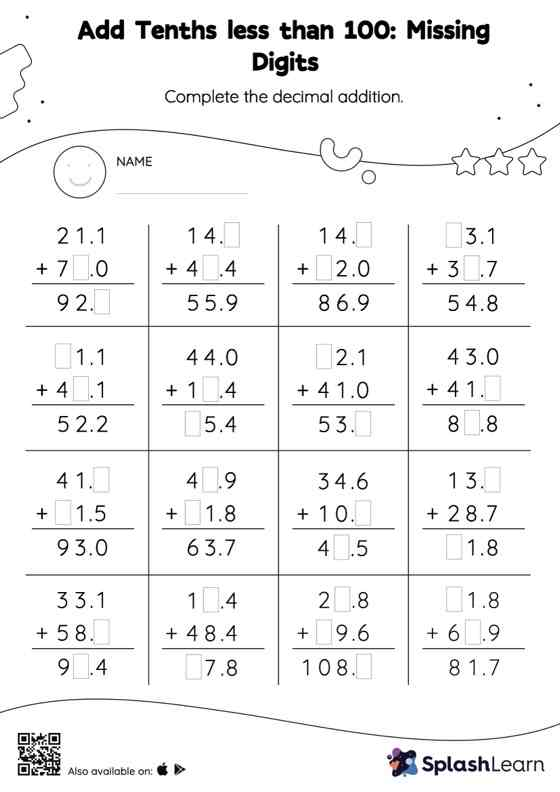# Add Tenths less than 100: Missing Digits Worksheet

Home > Add Tenths less than 100: Missing DigitsIn this worksheet, students add decimals by aligning the decimal points and using zero as a placeholder. They then use the relationship between addition and subtraction to find the missing number in the add tenths less than 100 worksheet. As the worksheet uses the column method, it is helpful in getting students toward higher accuracy, especially with bigger numbers and in scenarios where regrouping is required.